Refer to our Texas Go Math Grade 5 Answer Key Pdf to score good marks in the exams. Test yourself by practicing the problems from Texas Go Math Grade 5 Module 1 Assessment Answer Key.

Vocabulary

Concepts and Skills

Vocabulary
Commutative Property
Distributive Property
inverse operation
thousandth

Choose the best term from the box.

Question 1.
The ___________ states that multiplying a sum by a number is the same as multiplying each addend In the sum by the number and then adding the products.
We know that,
The “Distributive Property” states that multiplying a sum by a number is the same as multiplying each addend In the sum by the number and then adding the products
Hence, from the above,
We can conclude that
The best term that is suitable for the given statement is: Distributive Property

Question 2.
If one hundredth is divided Into ten equal parts, each part is one ____________
We know that,
If one hundredth is divided Into ten equal parts, each part is one “thousandth”
Hence, from the above,
We can conclude that
The best term that is suitable for the given statement is: Thousandth

Concepts and Skills

Write the value of the underlined digit.

Question 3.
6.54
The given decimal number is: 6.54
Now,
The representation of the given number in the place value chart is:Hence, from the above,
We can conclude that
The value of the underlined digit in the given number is: 4 hundredths

Question 4.
0.837
The given decimal number is: 0.837
Now,
The representation of the given number in the place value chart is: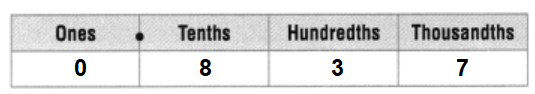Hence, from the above,
We can conclude that
The value of the underlined digit in the given number is: 8 tenths

Question 5.
8.702
The given decimal number is: 8.702
Now,
The representation of the given number in the place value chart is: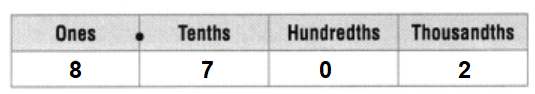Hence, from the above,
We can conclude that
The value of the underlined digit in the given number is: 2 thousandths

Question 6.
9.173
The given decimal number is: 9.173
Now,
The representation of the given number in the place value chart is: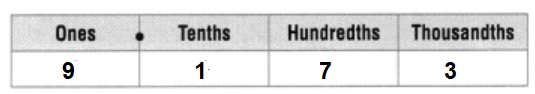Hence, from the above,
We can conclude that
The value of the underlined digit in the given number is: 9 ones

Compare. Write <, >, or =,

Question 7.
6.526.520
The given decimal numbers are: 6.52 and 6.520
Now,
From the given numbers,
We can observe that
All the place-values are the same
Hence, from the above,
We can conclude that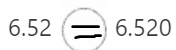Question 8.
3.5893.598
The given decimal numbers are: 3.589 and 3.598
Now,
We know that,
The place-value of “Hundredths” is greater than the place-value of “Thousandths”
Hence,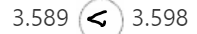Question 9.
8.4638.483
The given decimal numbers are: 8.463 and 8.483
Now,
We know that,
The place-value of “Hundredths” is greater than the place-value of “Thousandths”
Hence,Write the place value of the underlined digit. Round each number to the place of the underlined digit.

Question 10.
0.724
The given decimal number is: 0.724
Now,
The representation of the given number in the place value chart is: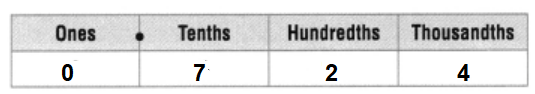So,
From the given place value chart,
The place value of 7 is: 7 Tenths
So,
The rounded number that is to the place of the underlined digit is: 0.7
Hence, from the above,
We can conclude that
The place value of 7 is: 7 Tenths
The rounded number that is to the place of the underlined digit is: 0.7

Question 11.
2.576
The given decimal number is: 2.576
Now,
The representation of the given number in the place value chart is: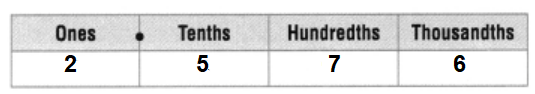So,
From the given place value chart,
The place value of 2 is: 2 ones
So,
The rounded number that is to the place of the underlined digit is: 2.6
Hence, from the above,
We can conclude that
The place value of 2 is: 2 ones
The rounded number that is to the place of the underlined digit is: 2.6

Question 12.
4.769
The given decimal number is: 4.769
Now,
The representation of the given number in the place value chart is:So,
From the given place value chart,
The place value of 6 is: 6 Hundredths
So,
The rounded number that is to the place of the underlined digit is: 4.8
Hence, from the above,
We can conclude that
The place value of 6 is: 6 Hundredths
The rounded number that is to the place of the underlined digit is: 4.8

Complete the equation, and tell which property you used.

Question 13.
8 × (14 + 7) = ________ + (8 × 7)
The given multiplication expression is:
8 × (14 + 7) = ________ + (8 × 7)
Now,
We know that,
The “Distributive Property” states that multiplying a sum by a number is the same as multiplying each addend In the sum by the number and then adding the products
So,
The completed equation is:
8 × (14 + 7) = (8 × 14) + (8 × 7)
Hence, from the above,
We can conclude that
The completed equation is:
8 × (14 + 7) = (8 × 14) + (8 × 7)
The property used is: Distributive Property

Question 14.
7 + (8 + 12) = ___________ + 12
7 + (8 + 12) = ___________ + 12
Now,
We know that,
According to the Associative Property of Addition,
If the grouping of addends changes, the sum stays the same
So,
The completed equation is:
7 + (8 + 12) = (7 + 8) + 12
Hence, from the above,
We can conclude that
The completed equation is:
7 + (8 + 12) = (7 + 8) + 12
The property used is: Associative Property of Addition

Find the sum or difference.

Question 15.
$14.85 –$4.63
The given numbers are: $14.85 and$4.63
Now,
By using the Long Subtraction,Hence, from the above,
we can conclude that
The result for the given subtraction of numbers is: $10.22 Question 16. 12.325 + 8.274 Answer: The given numbers are: 12.235 and 8.274 So, By using the Long Addition,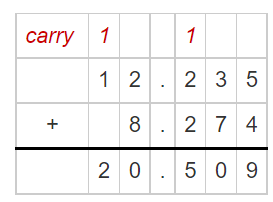Hence, from the above, we can conclude that The result for the given addition of numbers is: 20.509 Question 17. 7.423 – 5.15 Answer: The given numbers are: 7.423 and 5.15 So, By using the Long Subtraction,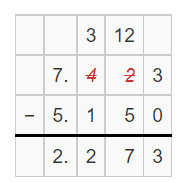Hence, from the above, we can conclude that The result for the given subtraction of numbers is: 2.273 Fill in the bubble completely to show your answer. Question 18. Maya uses a rope to make decorative necklaces for her friends. She has a total of 3.75 yards of rope. If she uses 0.48 yards of rope to make a necklace for her friend, how much rope does Maya have left? A. 4.13 yards B. 3.27 yards C. 3.17 yards D. 4.23 yards Answer: It is given that Maya uses a rope to make decorative necklaces for her friends. She has a total of 3.75 yards of rope. If she uses 0.48 yards of rope to make a necklace for her friend So, The length of rope does Maya have left = 3.75 – 0.48 = 3.27 yards Hence, from the above, We can conclude that The length of the rope does Maya have left is: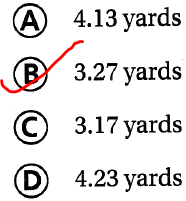Question 19. Stacy has$75 in her account. On Monday, she deposits $19.75 she earned babysitting. If Stacy buys a new pair of shoes for$40.35, how much money does Stacy have left in her account?
A. $40 B.$95
C. $55 D.$35
It is given that
Stacy has $75 in her account. On Monday, she deposits$19.75 she earned babysitting. If Stacy buys a new pair of shoes for $40.35 So, The amount of money does Stacy has left in her account =$75 + $19.75 –$40.35
= $54.4 ≈$55
Hence, from the above,
We can conclude that
The amount of money does Stacy has left in her account is:Question 20.
A honeybee can fly up to 2.548 meters per second. Which choice shows that number in expanded form?
A. 2 + 5.4 + 0.008
B. 2 + 0.5 + 0.48
C. 2 + 0.5 + 0.04 + 0.008
D. 2 + 0.5 + 0.04 + 0.08
It is given that
A honeybee can fly up to 2.548 meters per second
So,
The Expanded form of 2.548 is:
2 + 0.5 + 0.04 + 0.008
Hence, from the above,
We can conclude that
The choice that shows the given number in the expanded form is: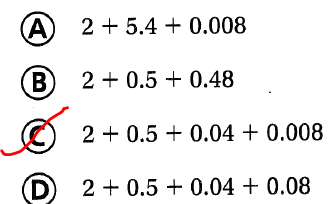Question 21.
Jan ran 1.256 miles on Monday, 1.265 miles on Wednesday, and 1.268 miles on Friday. What were her distances from greatest to least?
A. 1.268 miles, 1.256 miles, 1.265 miles
B. 1.268 miles, 1.265 miles, 1.256 miles
C. 1.265 miles, 1.256 miles, 1.268 miles
D. 1.256 miles, 1.265 miles, 1.268 miles
It is given that
Jan ran 1.256 miles on Monday, 1.265 miles on Wednesday, and 1.268 miles on Friday
Now,
We know that,
The place values of “Tenths” is greater than “Hundredths” is greater than “Thousandths”
So,
The order of the given numbers from the greatest to the least is:
1.268, 1.265, and 1.256
Hence, from the above,
We can conclude that
The order of Jan’s distances from the greatest to the least is: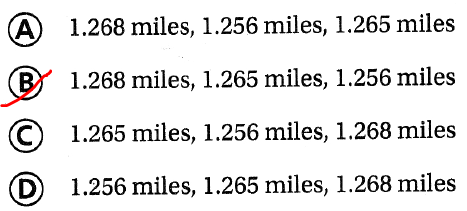Scroll to Top